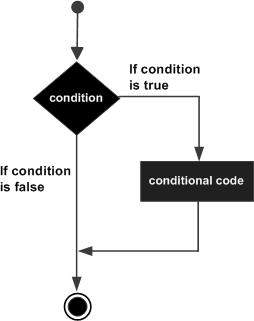# VBScript If Statement

An If statement consists of a Boolean expression followed by one or more statements. If the condition is said to be True, the statements under If condition(s) are Executed. If the Condition is said to be False, the statements after the If loop are executed.

## Syntax

The syntax of an If statement in VBScript is −

```If(boolean_expression) Then
Statement 1
.....
.....
Statement n
End If
```

## Flow Diagram## Example

```<!DOCTYPE html>
<html>
<body>
<script language = "vbscript" type = "text/vbscript">
Dim a : a = 20
Dim b : b = 10

If a > b Then
Document.write "a is Greater than b"
End If

</script>
</body>
</html>
```

When the above code is executed, it produces the following result −

```a is Greater than b
```
vbscript_decisions.htm